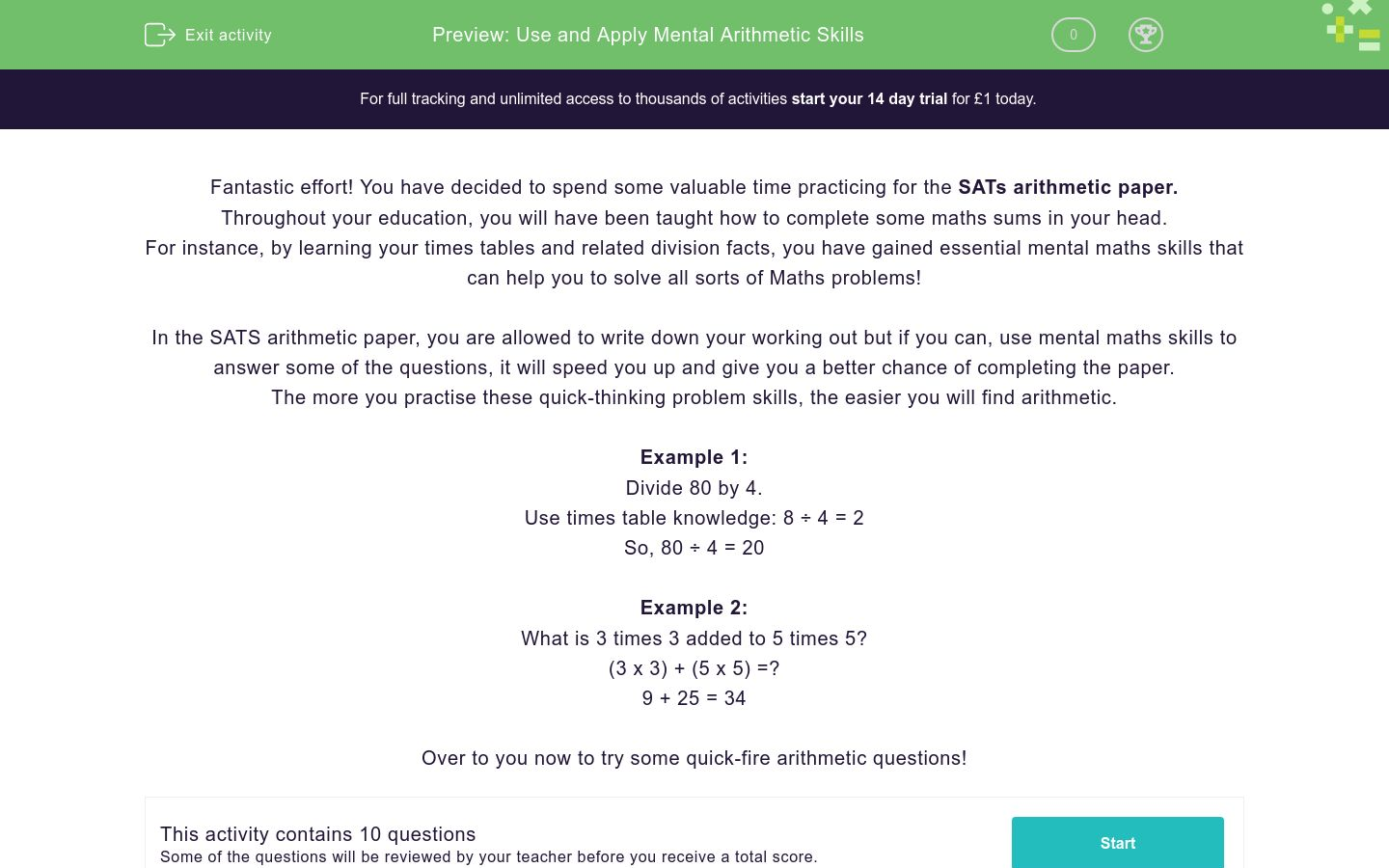# Use and Apply Mental Arithmetic Skills

In this worksheet, students will practise key mental maths skills in preparation for the SATs arithmetic paper. This worksheet will guide them through specific strategies to enable them to plan how to solve problems mentally.Key stage:  KS 2

Curriculum topic:   Exam-style Questions: SATs Maths

Curriculum subtopic:   Exam-Style Questions: Mental Maths

Difficulty level:### QUESTION 1 of 10

Fantastic effort! You have decided to spend some valuable time practicing for the SATs arithmetic paper.

Throughout your education, you will have been taught how to complete some maths sums in your head.

For instance, by learning your times tables and related division facts, you have gained essential mental maths skills that can help you to solve all sorts of Maths problems!

In the SATS arithmetic paper, you are allowed to write down your working out but if you can, use mental maths skills to answer some of the questions, it will speed you up and give you a better chance of completing the paper.

The more you practise these quick-thinking problem skills, the easier you will find arithmetic.

Example 1:

Divide 80 by 4.

Use times table knowledge: 8 ÷ 4 = 2

So, 80 ÷ 4 = 20

Example 2:

What is 3 times 3 added to 5 times 5?

(3 x 3) + (5 x 5) =?

9 + 25 = 34

Over to you now to try some quick-fire arithmetic questions!

826 + 300 = ?

1026

1126

926

526

1054 - 100 = ?

954

1154

854

1004

828 + 200 = ?

2026 - 100 = ?

5.4 + 5.03 = ?

10.40

10.43

10.14

10.03

3.2 - 1.1 = ?

2.0

1.9

1.8

2.1

97 x 3 = ?

758 x 10 = ?

Match each calculation with its correct answer...

## Column B

3.23 ÷ 10 =
4560
45.6 x 100 =
0.323
87.9 ÷ 100 =
0.879
2.28 x 10 =
22.8

314 - 80 = ?

• Question 1

826 + 300 = ?

1126
EDDIE SAYS
Strong start if you chose 1126! A quick method to answer this one is to start by focusing only on the hundreds digit. Add 300 to 800 = 1100. Then add the 26 on. 1100 + 26 = 1126.
• Question 2

1054 - 100 = ?

954
EDDIE SAYS
How did you do? This time we had to subtract 100. Focus on the thousands column, to begin with: 1000 - 100 = 900. Then add the 54 back on. 900 + 54 = 954. This great work- keep it up.
• Question 3

828 + 200 = ?

1028
EDDIE SAYS
Did you reach the answer is 1028? Focus on the hundreds column, 800 + 200 = 1000 Add the 28 back on = 1000 + 28 = 1028. Great place-value knowledge helps you to answer these kinds of questions quickly and correctly.
• Question 4

2026 - 100 = ?

1926
EDDIE SAYS
How are you doing? This time we had to subtract and find the answer 1926. Begin by subtracting 100 from 2000 = 1900. Then add the 26 back on. 1900 + 26 = 1926.
• Question 5

5.4 + 5.03 = ?

10.43
EDDIE SAYS
Did you get the hang of it? 5.4 + 5.03 = 10.43 The quickest way to solve this one mentally is to use partitioning: 5 + 5 = 10 0.4 + 0.03 = 0.43 10 + 0.43 = 10.43 If you are unsure in the test, quickly jot down the sum and check it using the column addition method.
• Question 6

3.2 - 1.1 = ?

2.1
EDDIE SAYS
Did you manage to spot that the answer is 2.1? To solve this one mentally, complete these steps using partitioning: 3 - 1 =2 0.2 - 0.1 = 0.1 Add the answers back together; 2 + 0.1 = 2.1 You're more than halfway through this activity- keep it up!
• Question 7

97 x 3 = ?

291
EDDIE SAYS
Did you find the answer 291? A quick way to solve this one is: 90 x 3 = 270 7 x 3 = 21 270 + 21 = 291.
• Question 8

758 x 10 = ?

7580
EDDIE SAYS
Brilliant work if you wrote 7580. You should be able to multiply by 10 mentally. Remember to move all of the digits, one place to the left.
• Question 9

Match each calculation with its correct answer...

## Column B

3.23 ÷ 10 =
0.323
45.6 x 100 =
4560
87.9 ÷ 100 =
0.879
2.28 x 10 =
22.8
EDDIE SAYS
Phew, that was a challenge! All of these sums should have been completed mentally using place value knowledge. Take your time to think carefully about how many places each digit should move and whether to move the digit to the left or the right. You've only got one question left!
• Question 10

314 - 80 = ?

234
EDDIE SAYS
Did you manage to get the answer of 234? A quick way to solve this mentally is to round 80 up to 100 and subtract: 314 - 100 = 214 Then add 20 back on 214 + 20 = 234 Fantastic effort- you have now completed another activity and are more prepared for the SATs arithmetic test.